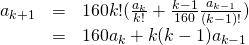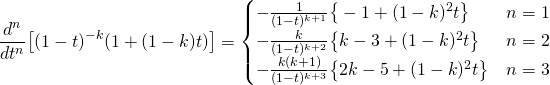# recurrence relation of 2nd-order

The following is a purely computational; including a process of converting a generating function to its formal power series relations. This can be also seen as an example of recurrence relation at the 2nd-order with indices coefficient.

Observe a case of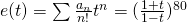To determine the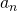, notice that the k-derivative has a form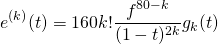where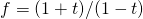and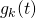is a monic polynomial of degree (k-1) with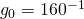.

By a calculation, one can see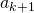has an inductive formula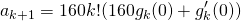And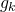has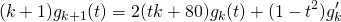Combining these formula, we have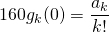First fewand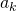are as follows.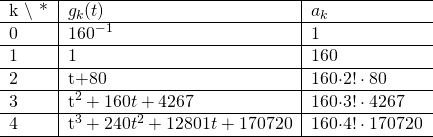By close look at the first-order term of,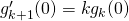holds. Thus there we have recurrence relation determined solely by means ofitself